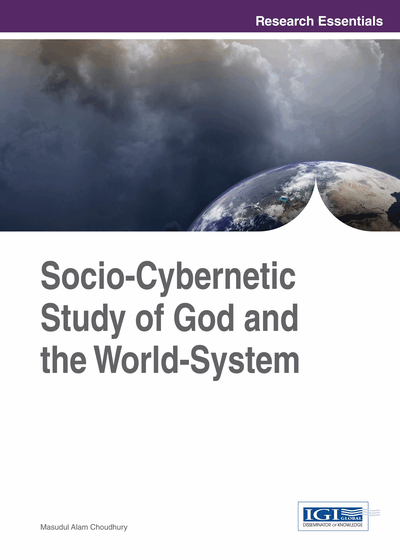# Productivity Analysis in Ethically Induced Financing Environment: A Case Study of Indonesian Islamic Banks

Copyright: © 2014 |Pages: 27
DOI: 10.4018/978-1-4666-4643-8.ch007
OnDemand PDF Download:
Available
\$37.50
No Current Special Offers

## Abstract

This chapter carries further the content of Chapter 6 to the measurement of an ethically value-induced index of productivity. A learning-form of the endogenously ethics-induced production function is formulated. This is empirically simulated for the case of Islamic banks in Indonesia that claim to introduce the moral and ethical elements of the Islamic Law in the affairs of finance and banking. Inferences are drawn for instructive purposes in the area of introducing the moral and ethical law in the study of business affairs, which is a significant subsystem of the grand world-system.
Chapter Preview
Top

## Background: The Neoclassical Impossibility In Measuring Productivity And Efficiency In Social Financial Environment

In common parlance, the term, ‘factor productivity’ means the ratio of output to specific factor inputs. In the input-output sense, productivity can also be measured by the input-output technical coefficients of production. The technical input-output coefficient aij is defined by the ratio (technical coefficient) aij = xij/xj, meaning the intensity with which the output of ith sector (or project, source) is used as input to produce a unit of total output in the jth sector (project, source), i,j = 1,2,..,n.

In the case of an endogenous technologically induced factor input over time, x1(t) [hence x2(t)], such a time-dynamic factor-productivity is explained by the expression (Solow, 1980, pp. 33-38),

Q(t)/x1(t) = x1(t)αf(k(t))/x1(t) = f(k(t)).x1(α-1), k = x2(t)/x1(t).

α > 1 denotes the degree of the homogenous production function, Q(t) = f(x1(t),x2(t)), which stands for the production function in the factor inputs x1(t) and x2(t). α > 1 equivalently also denotes the degree of economy of scale of production in the formal production function.

The above form of the neoclassical production function and the underlying input production isoquants necessitate existence of well-defined formal expressions for these cases. Accordingly, the neoclassical economic theory implication is that, there will abide marginal rate of substitution between the production outputs, as between different competing sectors and projects. There will likewise be opportunity costs of substitution between the factor inputs along their production isoquants. The same implication is borne out by the assumption of smoothly defined production possibility curves and isoquants.

Scarcity of productive resources, competition between opposites in outputs and inputs, and the concurrent need for smooth production possibility surfaces define the opportunity costs of substitution between outputs and inputs. The resulting definition of the production function in terms of outputs and inputs are permanently premised on the above axiomatic premises of neoclassical theory of production and cost. The resulting productivity and efficiency analyses are then carried out.

Yet the same axiomatic premises are untenable when participatory financing is studied by its relevant different models of fuzzy joint production functions and social contracts based on complementary relations between the factor inputs and output distribution by productive linkages across sectors, regions, and other socioeconomic environments. The idea underlying complex forms of production relations is that there exist extensive linkages between the production menus, their inputs, other outputs, technology, and sustainable development of the firm’s structure and organizational behavior. Such organic interrelations result in extensive fields of circular causation relations, backward and forward. A joint production function and a dynamic form of input-output system of learning relations between complementary interactive possibilities establish a financial-productive social nexus (Choudhury, 2010).

In the neoclassical case, the outputs such as all of the paired types of variables {yi(t) yj(t)}, and thereby all of yij(t)’s, i,j = 1,2,..n; likewise all of {x1(t),x2(t)}, exist as marginal substitutes of each other. Hence the production possibility surfaces and the production isoquants so formed remain smoothly convex and concave to the origin, respectively. Such a result is premised on the assumptions of marginal substitution and smooth production surfaces and isoquants mentioned above. They then lead to a production cost line and price relatives that are deterministic or are valued probabilistically to obtain expected values for the production indicators. It is therefore, logical that the relations between outputs and inputs in various sectors and kinds, must remain observable as datum. Consequently, productivity as a computed measure related to such assumedly well-determined production menus and isoquants related to minimum average cost is a deterministic or probabilistically quantifiable economic indicator.

## Complete Chapter List

Search this Book:
Reset